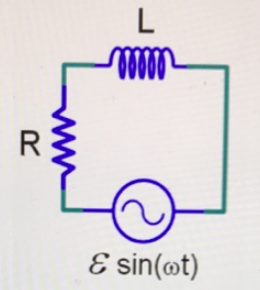# Problem: A RL circuit is driven by an AC generator as shown in the figure.The phase difference between the CURRENT through the resistor and inductor ___________.A. is always zeroB. is always 90°C. depends on the value of L and RD. depends on L, R, and the generator voltageBriefly explain you reasoning.

###### FREE Expert Solution

Current:

$\overline{){\mathbf{i}}{\mathbf{=}}\frac{\mathbf{v}}{\mathbf{R}}}$

The phase difference between the inductor and the resistor remains constant at 90°.

Since the circuit is the same, it will be the same current.###### Problem Details

A RL circuit is driven by an AC generator as shown in the figure.The phase difference between the CURRENT through the resistor and inductor ___________.

A. is always zero

B. is always 90°

C. depends on the value of L and R

D. depends on L, R, and the generator voltage

Briefly explain you reasoning.